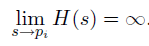Home | Tutorials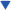| Projects| Construction Tips| Store

### Welcome To KenSeibert.Com Audio

What is a Pole

If you have been reading about filters at all, you have probably encountered wording like "2-pole low pass filter" or "4-pole high pass filter". If you were wondering just what a "Pole" is, then maybe this will answer your question.

The answer involves math. If you are not a fan of equations, there is not much we can do the explain. Below are two explanations. One is the simple version, the other is the right version. Albert Einstein once rightly said: "You should make things as simple as possible - bit no simpler!" In this case, the simple version still involves math, but stops at Algebra, and is too simple, but still gives an explanation.

Both explanations involve what is known as a Transfer Function. This is a mathematical way of expressing what a filter does. If x is the input, then F(x) is the output. When this function is graphed, there are some points where the function goes to zero (these are called 'Zeros"). There are other points where the function goes to infinity - these are called "Poles"

 Simple Version The transfer function of a filter can be thought of as a ratio of two polynomials. In your high school Algebra class you probably remember that a polynomial looks like this: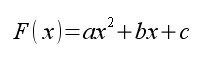A ratio of two polynomials would look like this: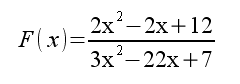Again, from Algebra, you remember that a polynomial can be factored in order to solve the equation. In this case the function above can be written as: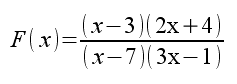The Numerator of the equation has two points where it goes to zero. If the numerator goes to zero, the entire function goes to zero. These two points are the 'Zeros" of the equation. Likewise, the denominator has two points where it goes to zero. If the denominator of a function goes to zero, the function goes to infinity. These points are called the "Poles" of the function. If there are two values of x where the denominator goes to zero, then the function is a 2-Pole function. In a filter's transfer function if there are two values where the result goes to infininty, the filter is called a 2-Pole filter. If there are 4 values where it goes to infinity, then it is a 4-Pole filter, and so on. Correct Version The transfer function of a filter is a differential equation of the complex variable s: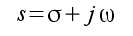For example:This can be written in a more general form as: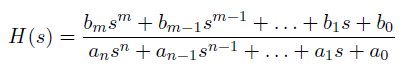Or factored as: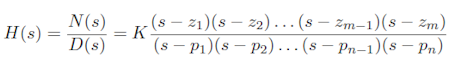From this we can see that the roots of the numerator polynomial N(s) are the zeros of the function and the roots of the denominator polynomial D(s) are the poles. This is due to the fact that as s approaches a root, D(s) approaches 0 and the equation becomes unbounded.